# The Runcitruncated 600-cell

The runcitruncated 600-cell is a member of the 120-cell/600-cell family of 4D uniform polytopes. Its surface consists of 2640 cells (120 rhombicosidodecahedra, 720 pentagonal prisms, 1200 hexagonal prisms, 600 truncated tetrahedra), 13440 polygons (2400 triangles, 7200 squares, 2400 hexagons, 1440 pentagons), 18000 edges, and 7200 vertices.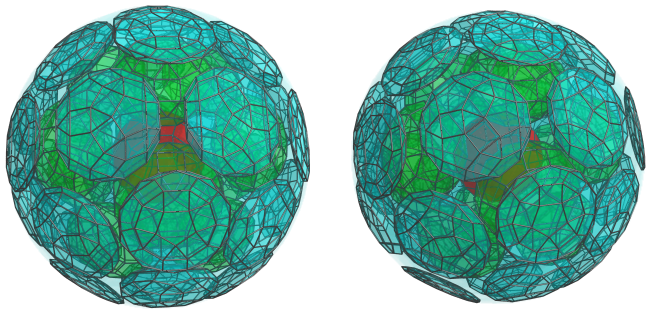It may be constructed by expanding the rhombicosidodecahedral cells of the cantellated 120-cell outwards radially, and filling in the gaps with pentagonal prisms, hexagonal prisms, and truncated tetrahedra.

## Structure

We shall explore the structure of the runcitruncated 600-cell using its orthogonal projections into 3D.

### First Layer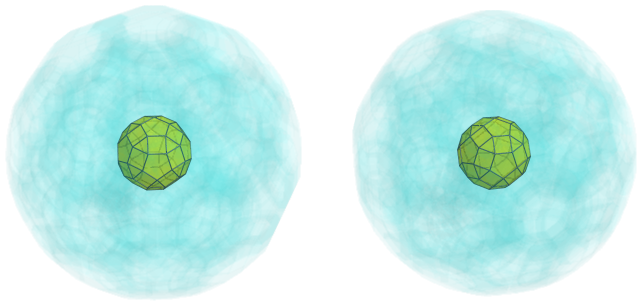This image shows the nearest rhombicosidodecahedron to the 4D viewpoint. For clarity, we have rendered the other cells in a light transparent colour, and only show the edges that lie on this cell. The cells that lie on the far side of the polychoron have been culled.

The pentagonal faces of this nearest cell are joined to 12 pentagonal prisms, as shown in the next image: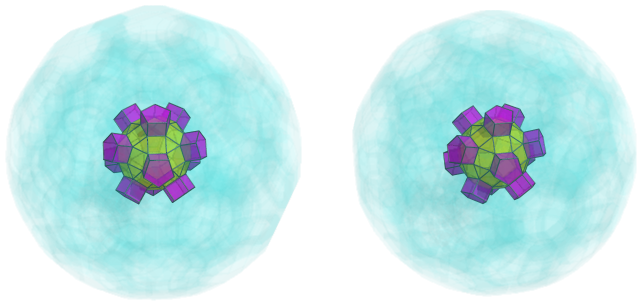The square faces of the nearest cell are joined to 30 hexagonal prisms: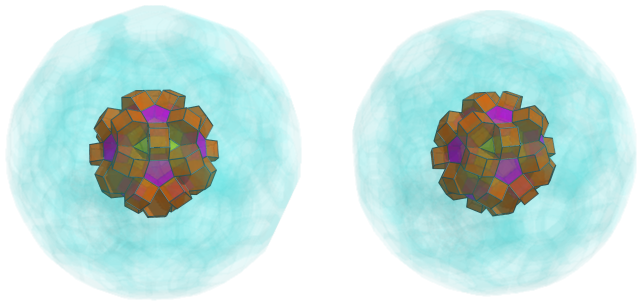The remaining triangular faces of the nearest cell are joined to 20 truncated tetrahedra: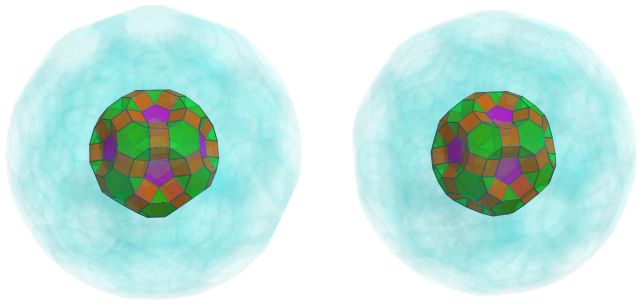### Second Layer

The 12 depressions seen in the previous image are where the next layer of 12 rhombicosidodecahedra are fitted: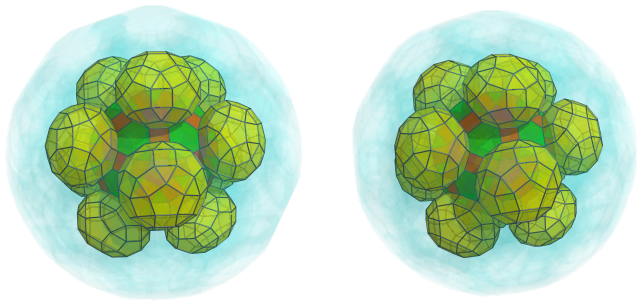These rhombicosidodecahedra are connected by a system of pentagonal prisms, which overlie the hexagonal prisms from the previous layer: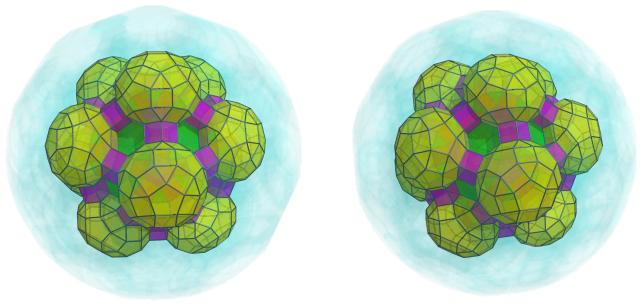There are 30 such pentagonal prisms in total.

Next, hexagonal prisms fill in the 20 gaps overlying the truncated tetrahedra from the previous layer: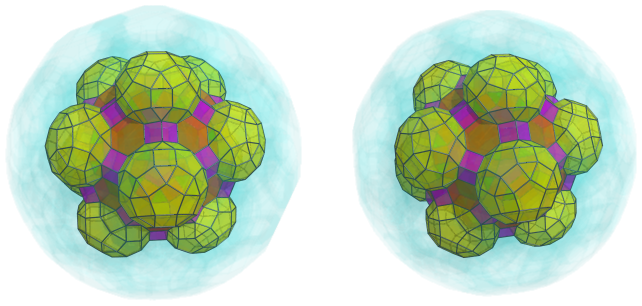### Third Layer

On top of the hexagonal prisms in the previous layer are another 20 truncated tetrahedra: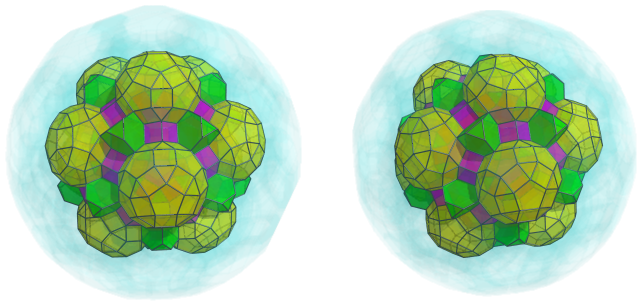These truncated tetrahedra are bridged by 30 pairs of hexagonal prisms that share an edge, for a total of 60 more hexagonal prisms: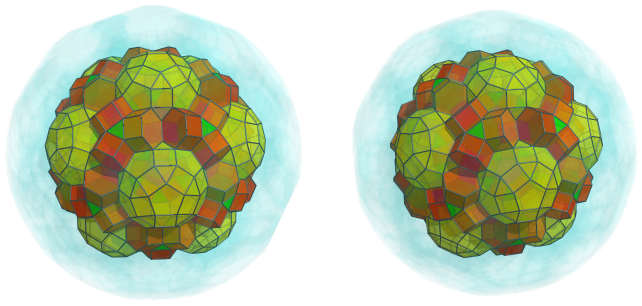Between each pair of hexagonal prisms are yet another 30 truncated tetrahedra: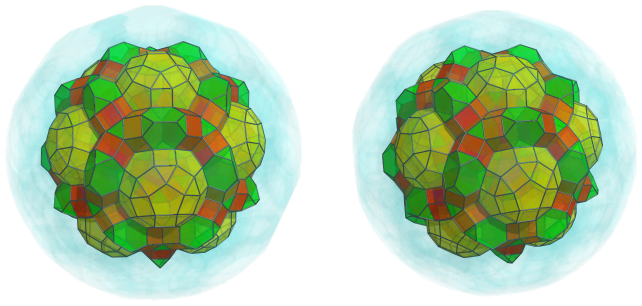There are also 60 pentagonal prisms in twelve rings of 5 each, encircling the rhombicosidodecahedral cells.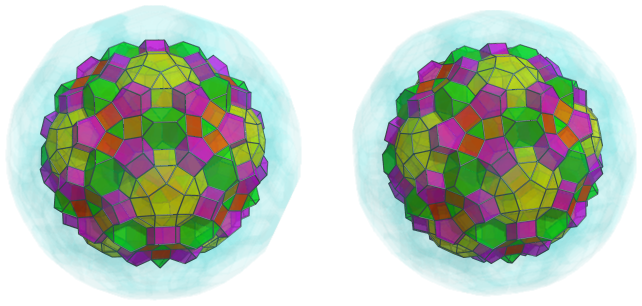These pentagonal prisms alternate with 60 hexagonal prisms: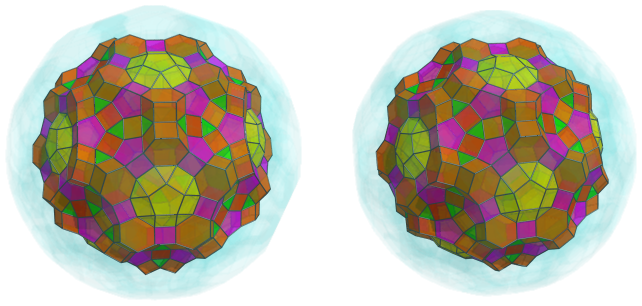### Fourth Layer

The bowl-shaped depressions that are starting to show are where another 20 rhombicosidodecahedra sit: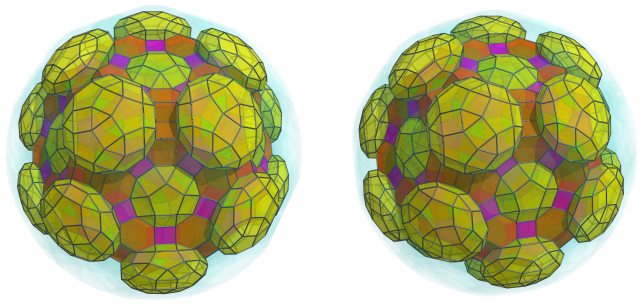These cells are connected to each other by 30 pentagonal prisms: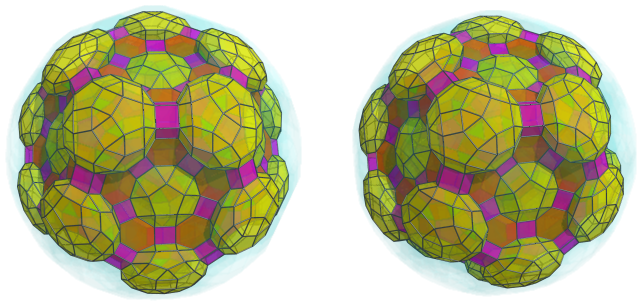Around the base of these cells are circles of truncated tetrahedra, 60 in total: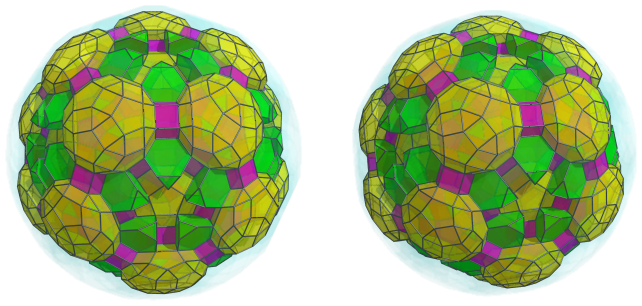These truncated tetrahedra alternate with 60 hexagonal prisms: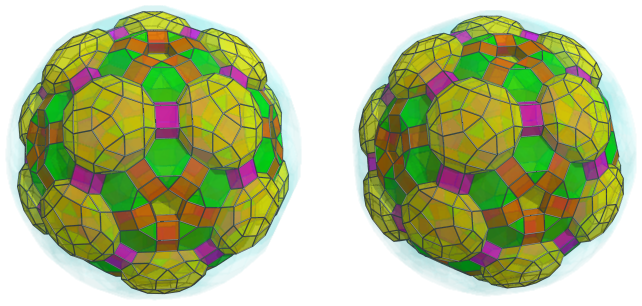It should be obvious now that at the center of these circles of truncated tetrahedra and hexagonal prisms are gaps that can be filled in by pentagonal prisms. There are 12 such pentagonal prisms in total: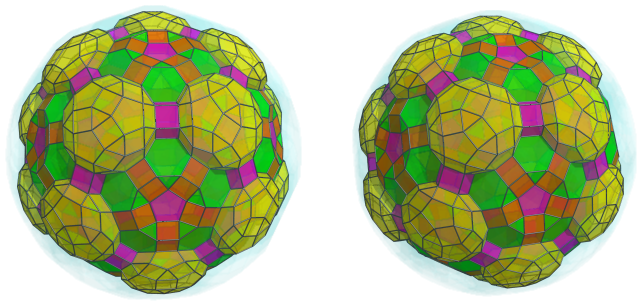### Fifth Layer

More pentagonal prisms overlie the circles of alternating hexagonal prisms and truncated tetrahedra; another 60 in total: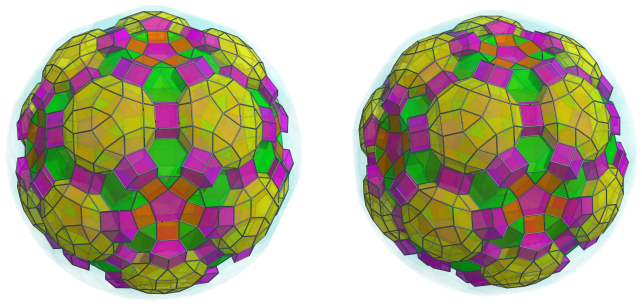These pentagonal prisms are part of another layer of alternating prisms, with 60 more hexagonal prisms between them: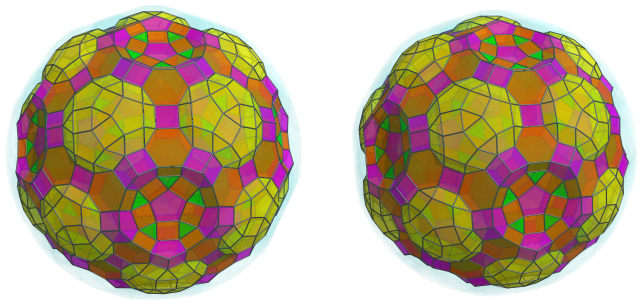The new depressions that are forming are where another layer of 12 rhombicosidodecahedra are situated: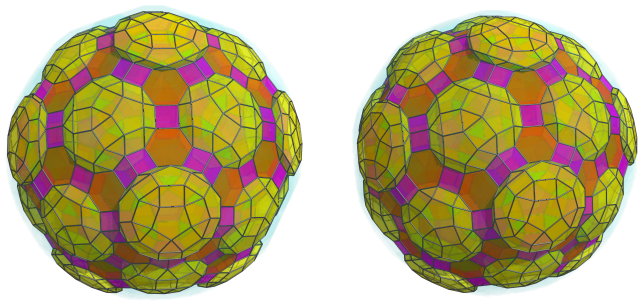### Sixth Layer

There are 60 more truncated tetrahedra that sit atop the 60 visible hexagonal prisms from the previous layer: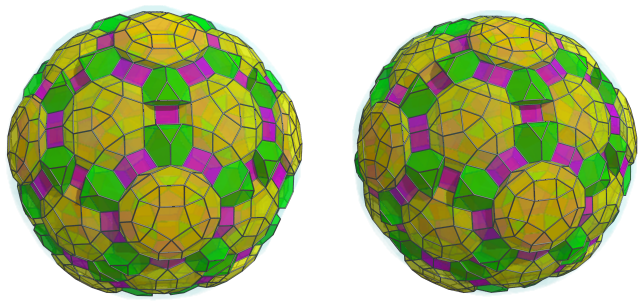Hexagonal prisms link 30 pairs of these truncated tetrahedra: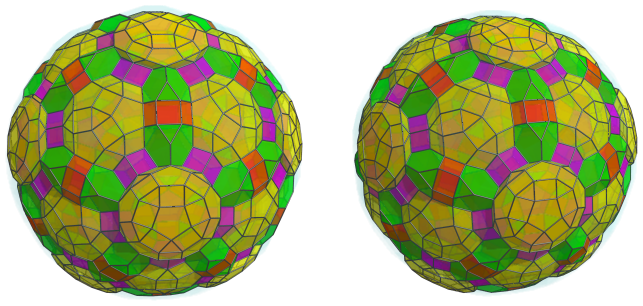The remaining pairs of truncated tetrahedra are bridged by pairs of hexagonal prisms sharing an edge. There are 60 such pairs, making a total of 120 more hexagonal prisms: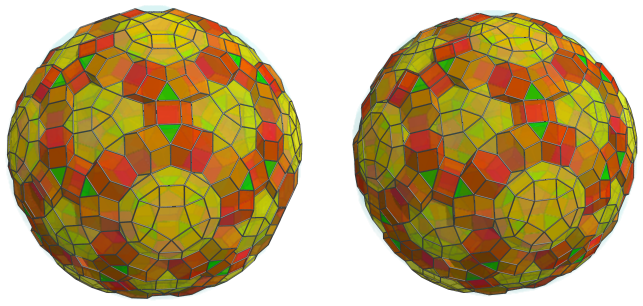### Seventh Layer

Straddling each of the previous pairs of hexagonal prisms are 60 more truncated tetrahedra: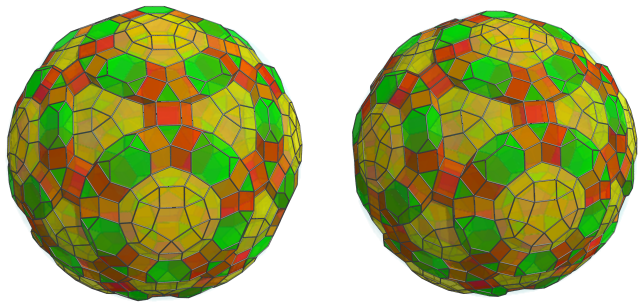The edges of these truncated tetrahedra touch a number of pentagonal prisms that overlie the exposed pentagonal faces of the rhombicosidodecahedra. There are 120 pentagonal prisms in total, 60 of which are distributed in circles of 5 around 12 of the rhombicosidodecahedra, and the other 60 in triplets over the other 20 rhombicosidodecahedra.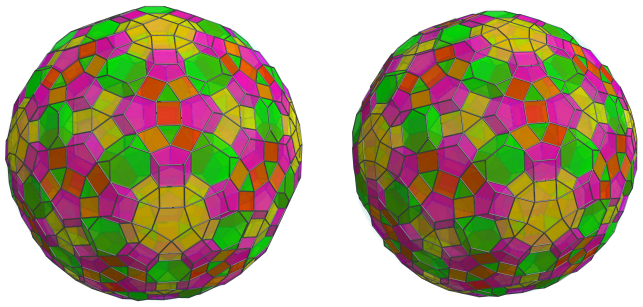Alternating with these pentagonal prisms are another 120 hexagonal prisms: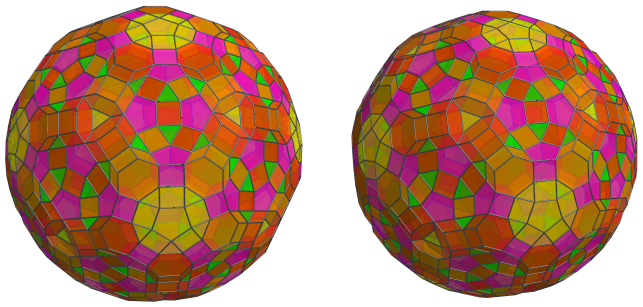Finally, another 20 truncated tetrahedra sit in the shallow depressions formed by triplets of hexagonal prisms: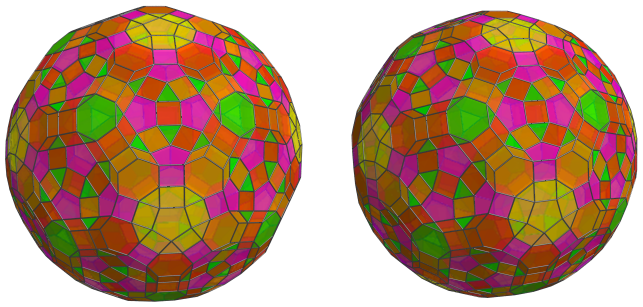These are all the cells of the runcitruncated 600-cell that lie on its near side, the side facing the 4D viewpoint. Past this point, we reach the cells on its “equator”: those that are seen from a 90° angle from the 4D viewpoint.

### The Equator

Now we come to the “equator” of the runcitruncated 600-cell. There are 30 rhombicosidodecahedral cells, as shown below: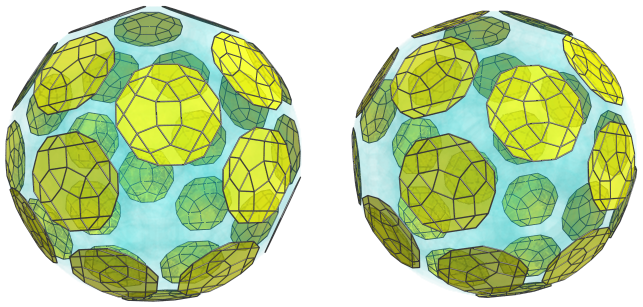For clarity, we have omitted the cells on the near side of the polytope that were shown earlier. These rhombicosidodecahedra appear flattened into dodecagons, because they are being seen from a 90° angle. In 4D, they are perfectly uniform rhombicosidodecahedra.

These cells are bridged to each other by 60 pentagonal prisms: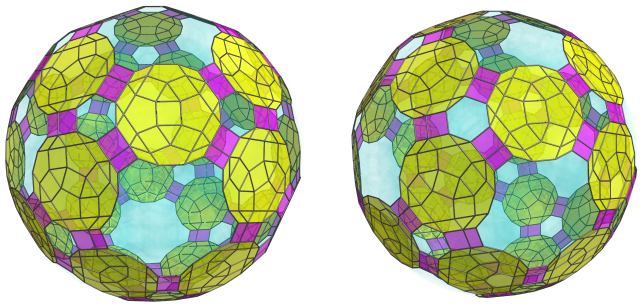These pentagonal prisms are being seen from a 90° angle, in a lateral orientation; hence they appear foreshortened into rectangles. In 4D, they are perfectly uniform pentagonal prisms.

These aren't the only pentagonal prisms that lie on the equator; there are another 12 of them in a different orientation that appear, from the 4D viewpoint, foreshortened into regular pentagons: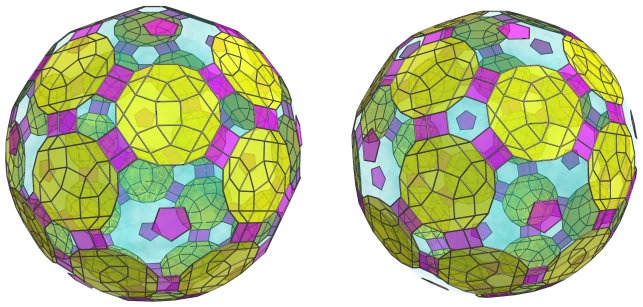Surrounding these 12 pentagonal prisms are 60 truncated tetrahedra, arranged in florets of 5 each: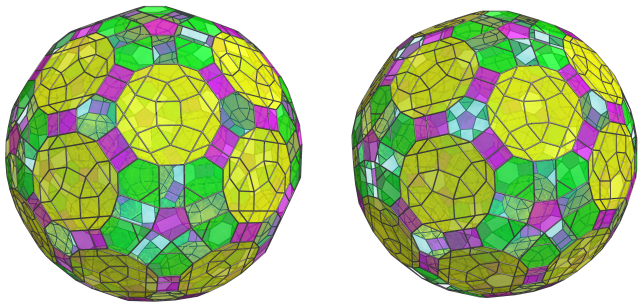These truncated tetrahedra have been foreshortened into irregular pentagons because they are being seen from 90° angle. They are perfectly uniform truncated tetrahedra in 4D.

Alternating with them are 60 hexagonal prisms: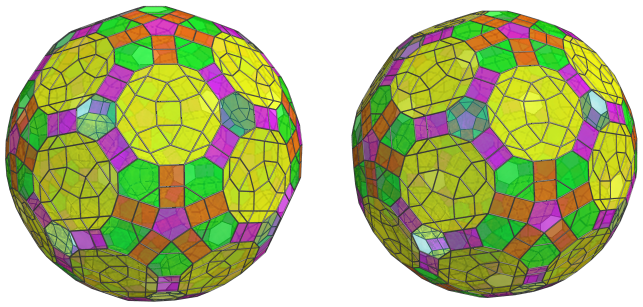These hexagonal prisms have been foreshortened into rectangles because they are being seen from a 90° angle. They aren't the only hexagonal prisms on the equator; there are another 20 of them in a different orientation that causes them to appear in projection as regular hexagons: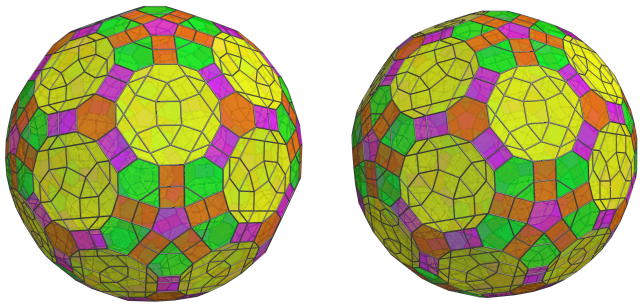These are all the cells that lie on the equator of the runcitruncated 600-cell. Past this point, we reach the far side of the polytope, where the arrangement of cells mirrors that of the near side that we have seen earlier, with the layers in reverse order.

### Summary

The following table summarizes the cell counts of each layer of the runcitruncated 600-cell:

Region Layer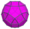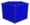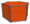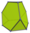Near side 1 1 12 30 20
2 12 30 20 0
3 0 60 60 + 60 = 120 20 + 30 = 50
4 20 30 + 12 = 42 60 60
5 12 60 60 0
6 0 0 30 + 120 = 150 60
7 0 120 120 60 + 20 = 80
Subtotal 45 324 560 270
Equator 30 60 + 12 = 72 60 + 20 = 80 60
Far side 7 0 120 120 80
6 0 0 150 60
5 12 60 60 0
4 20 42 60 60
3 0 60 120 50
2 12 30 20 0
1 1 12 30 20
Subtotal 45 324 560 270
Grand total 120 720 1200 600

## Coordinates

The coordinates of the runcitruncated 600-cell, centered on the origin and having edge length 2, are all permutations of coordinates and changes of sign of:

• (1, 1, φ3, 3+8φ)
• (1, 1, 1+4φ, 5+6φ)
• 2, 3+φ, φ5, φ5)
• (2+φ, 2+φ, 2+5φ, 4+5φ)
• (3φ, φ4, 2+5φ, 2+5φ)
• (1+3φ, 1+3φ, 1+5φ, φ5)

along with even permutations coordinates and all changes of sign of:

 (0, 1, 2+5φ, 5φ2) (0, φ2, 3+8φ, 2+φ) (0, φ3, 4+7φ, 3+φ) (0, 2φ2, 2+6φ, 4φ2) (0, 1+3φ, 3φ3, 4+3φ) (1, φ, 2φ4, 1+5φ) (1, 2, φ5, 4+5φ) (1, 2, 1+3φ, 4+7φ) (1, 2+φ, 2+6φ, φ5) (1, φ3, 5+6φ, 3+2φ) (1, 3φ, 2φ4, 3φ2) (1, 3φ2, 2+5φ, 4φ2) (1, 2φ3, φ5, 4+3φ) (φ, φ2, 2φ, 3+8φ) (φ, 2+φ, 3φ, 4+7φ) (φ, 3+2φ, φ5, 4φ2) (φ, φ4, 4+5φ, 4+3φ) (2, φ2, 3φ3, 2+5φ) (2, φ2, 5+6φ, φ4) (2, 2φ, 2φ4, 2φ3) (φ2, 3φ, 5+6φ, 2φ2) (φ2, 1+3φ, 5φ2, 3φ2) (φ2, 3φ2, 1+5φ, φ5) (2φ, 2+φ, 1+3φ, 5+6φ) (2φ, 3+2φ, 2+5φ, φ5) (2φ, 1+4φ, 4+5φ, 3φ2) (2+φ, φ3, 5φ2, 2φ3) (2+φ, 1+3φ, 2φ4, 3+2φ) (φ3, 3+φ, φ4, 2φ4) (φ3, 2φ2, 1+5φ, 4+5φ) (φ3, 3+2φ, 1+4φ, 3φ3) (φ3, 2φ3, 1+5φ, 2+5φ) (3φ, 1+4φ, φ5, 2φ3) (1+3φ, φ4, 1+4φ, 2+6φ)

where φ=(1+√5)/2 is the Golden Ratio.

Last updated 18 Jun 2019.